# Create KMunicate-Style Plots

The {KMunicate} package can be used to produce Kaplan-Meier plots in the style recommended following the KMunicate study by TP Morris et al. (2019).

In this vignette, we’ll see learn how to produce such a plot using the {KMunicate} package.

## Data

We will be using the `brcancer` dataset, which comes bundled with {KMunicate}:

``````data("brcancer", package = "KMunicate")
str(brcancer)
#> Classes 'tbl_df', 'tbl' and 'data.frame':    686 obs. of  14 variables:
#>  \$ id     : num  1 2 3 4 5 6 7 8 9 10 ...
#>  \$ hormon : num  0 1 1 1 0 0 1 0 0 0 ...
#>  \$ x1     : num  70 56 58 59 73 32 59 65 80 66 ...
#>  \$ x2     : num  2 2 2 2 2 1 2 2 2 2 ...
#>  \$ x3     : num  21 12 35 17 35 57 8 16 39 18 ...
#>  \$ x4     : num  2 2 2 2 2 3 2 2 2 2 ...
#>  \$ x5     : num  3 7 9 4 1 24 2 1 30 7 ...
#>  \$ x6     : num  48 61 52 60 26 0 181 192 0 0 ...
#>  \$ x7     : num  66 77 271 29 65 13 0 25 59 3 ...
#>  \$ rectime: num  1814 2018 712 1807 772 ...
#>  \$ censrec: num  1 1 1 1 1 1 0 0 1 0 ...
#>  \$ x4a    : num  1 1 1 1 1 1 1 1 1 1 ...
#>  \$ x4b    : num  0 0 0 0 0 1 0 0 0 0 ...
#>  \$ x5e    : num  0.698 0.432 0.34 0.619 0.887 ...
#>  - attr(*, "label")= chr "German breast cancer data"``````

## Single-Arm Plot

We start by creating a KMunicate-style plot for all study subjects. First, we load the package:

``````library(KMunicate)

Then, we fit a Kaplan-Meier curve using the `survfit` function from the {survival} package:

``````fit <- survfit(Surv(rectime, censrec) ~ 1, data = brcancer)
fit
#> Call: survfit(formula = Surv(rectime, censrec) ~ 1, data = brcancer)
#>
#>        n events median 0.95LCL 0.95UCL
#> [1,] 686    299   1807    1587    2030``````

Then, we need to define the horizontal axis of the plot. For instance, we can define 5 equally-spaced breaks between time zero and the largest observed time:

``````ts <- seq(0, max(brcancer\$rectime), length.out = 5)
ts
#>     0.00  664.75 1329.50 1994.25 2659.00``````

Finally, we can pass `fit` and `ts` to the `KMunicate()` function to simply obtain a plot:

``KMunicate(fit = fit, time_scale = ts)``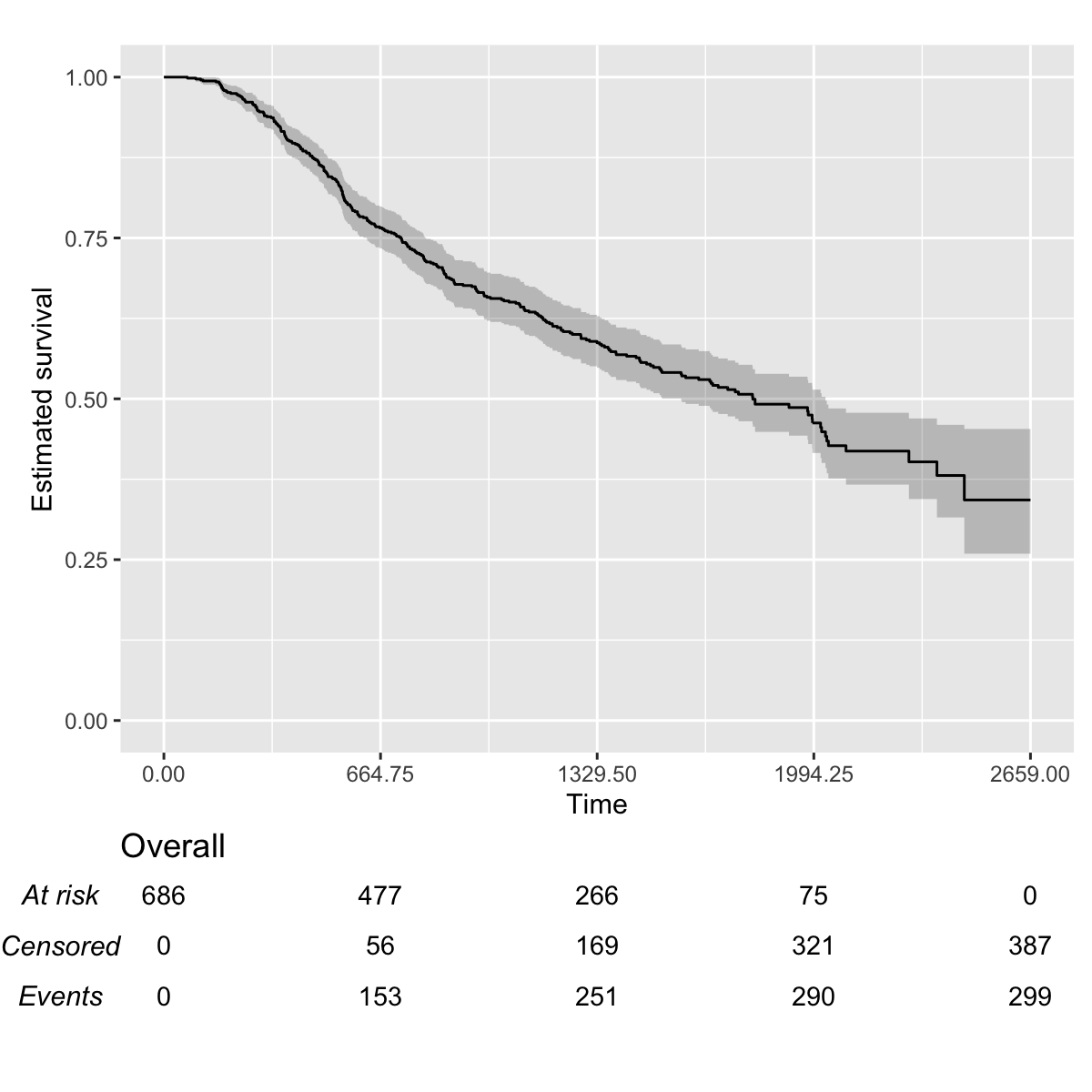## Multiple-Arms Plot

A multiple-arms plot will be automatically produced if the `survfit` object has covariates in it. For instance, if we fit the Kaplan-Meier estimator by treatment arm:

``````fit2 <- survfit(Surv(rectime, censrec) ~ hormon, data = brcancer)
fit2
#> Call: survfit(formula = Surv(rectime, censrec) ~ hormon, data = brcancer)
#>
#>            n events median 0.95LCL 0.95UCL
#> hormon=0 440    205   1528    1296    1814
#> hormon=1 246     94   2018    1918      NA``````

We will be using the same time scale as before (`ts`), and the call to `KMunicate()` is analogous:

``KMunicate(fit = fit2, time_scale = ts)``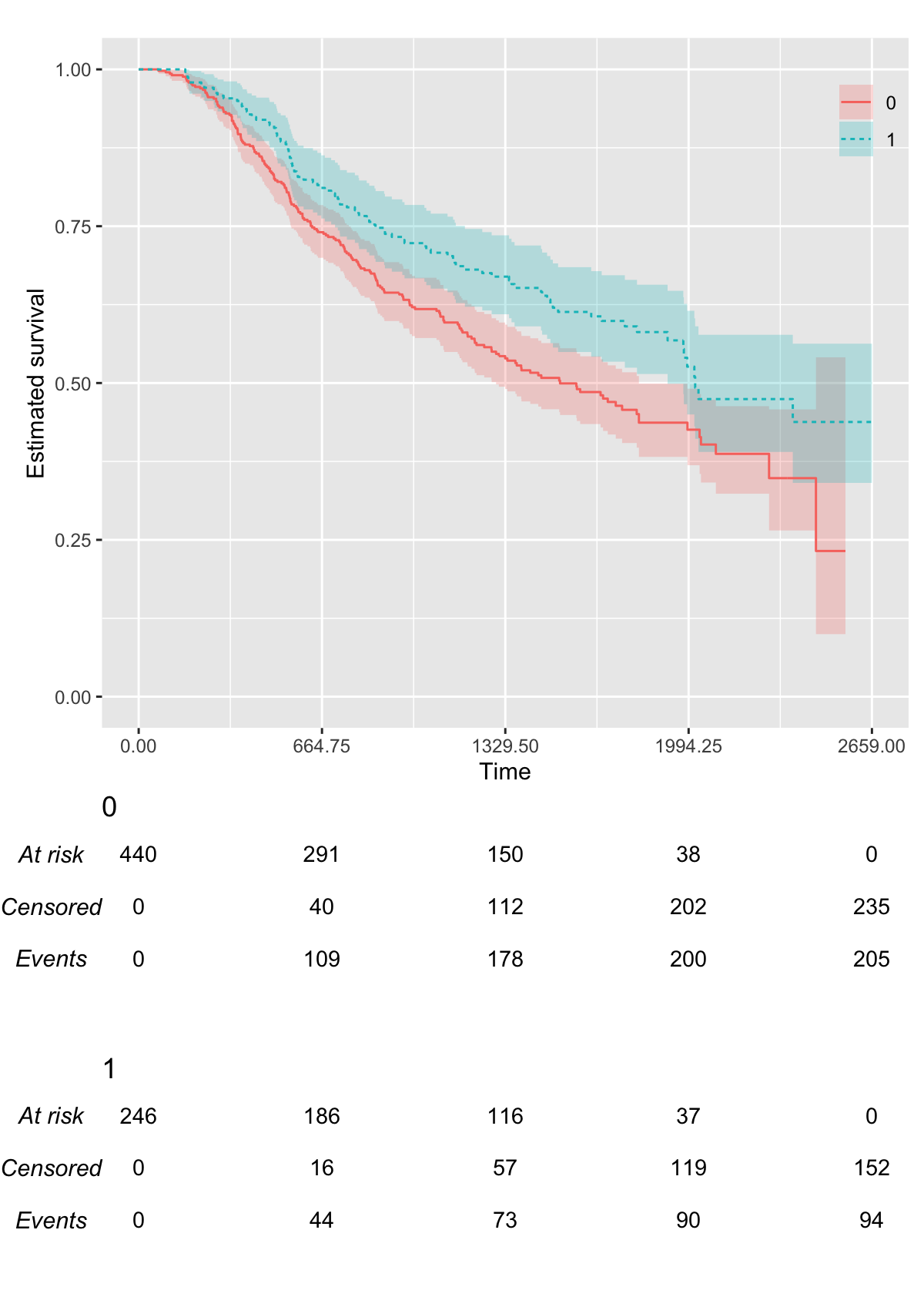## Customisation

The `KMunicate()` function contains a few options to customise the produced plot. First, we can pass a `ggplot2` theme to the `.theme` argument:

``KMunicate(fit = fit2, time_scale = ts, .theme = ggplot2::theme_minimal())``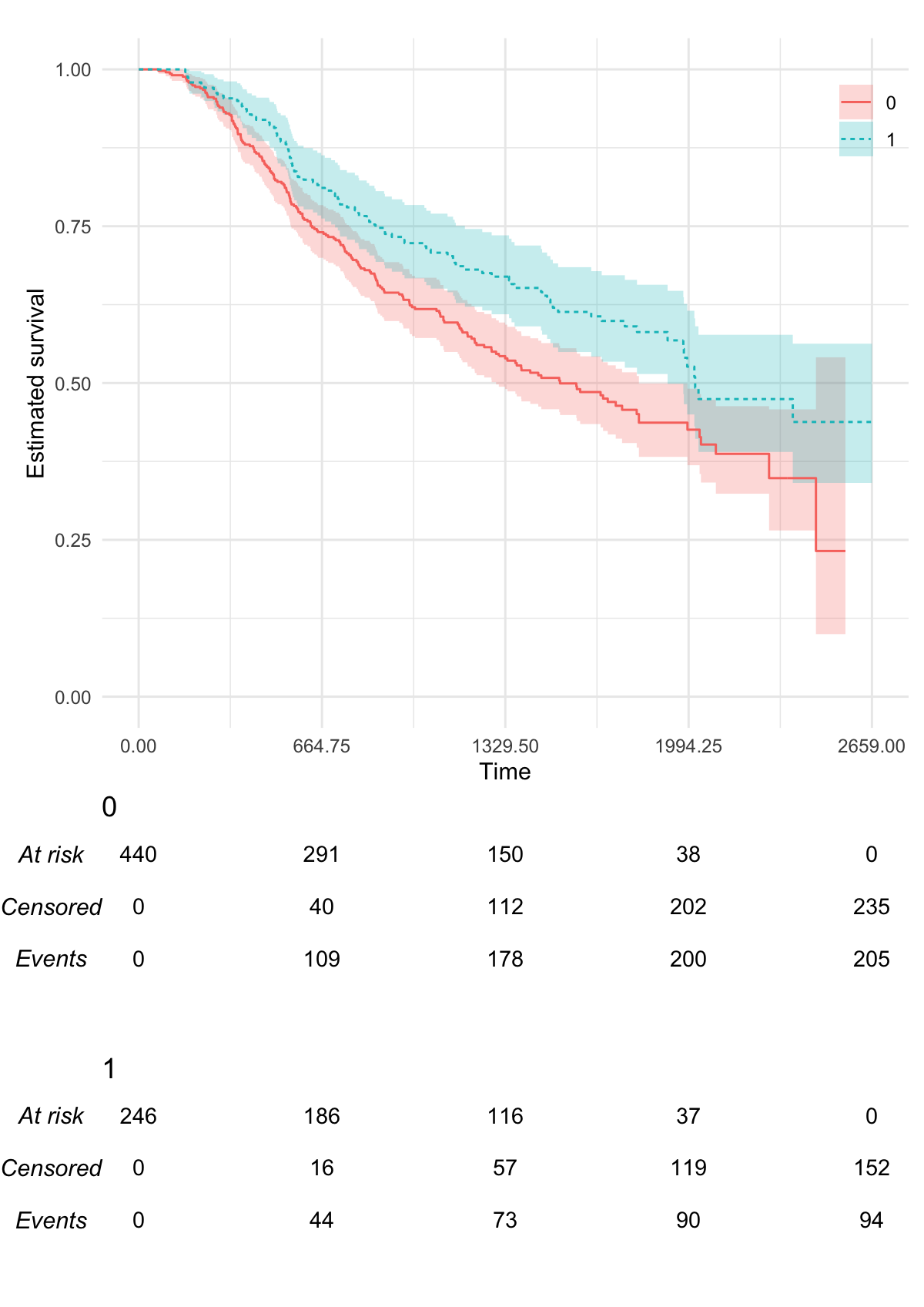We can also pass custom colour (and fill) scales:

``````KMunicate(
fit = fit2,
time_scale = ts,
.color_scale = ggplot2::scale_color_brewer(type = "qual", palette = "Set2"),
.fill_scale = ggplot2::scale_fill_brewer(type = "qual", palette = "Set2")
)``````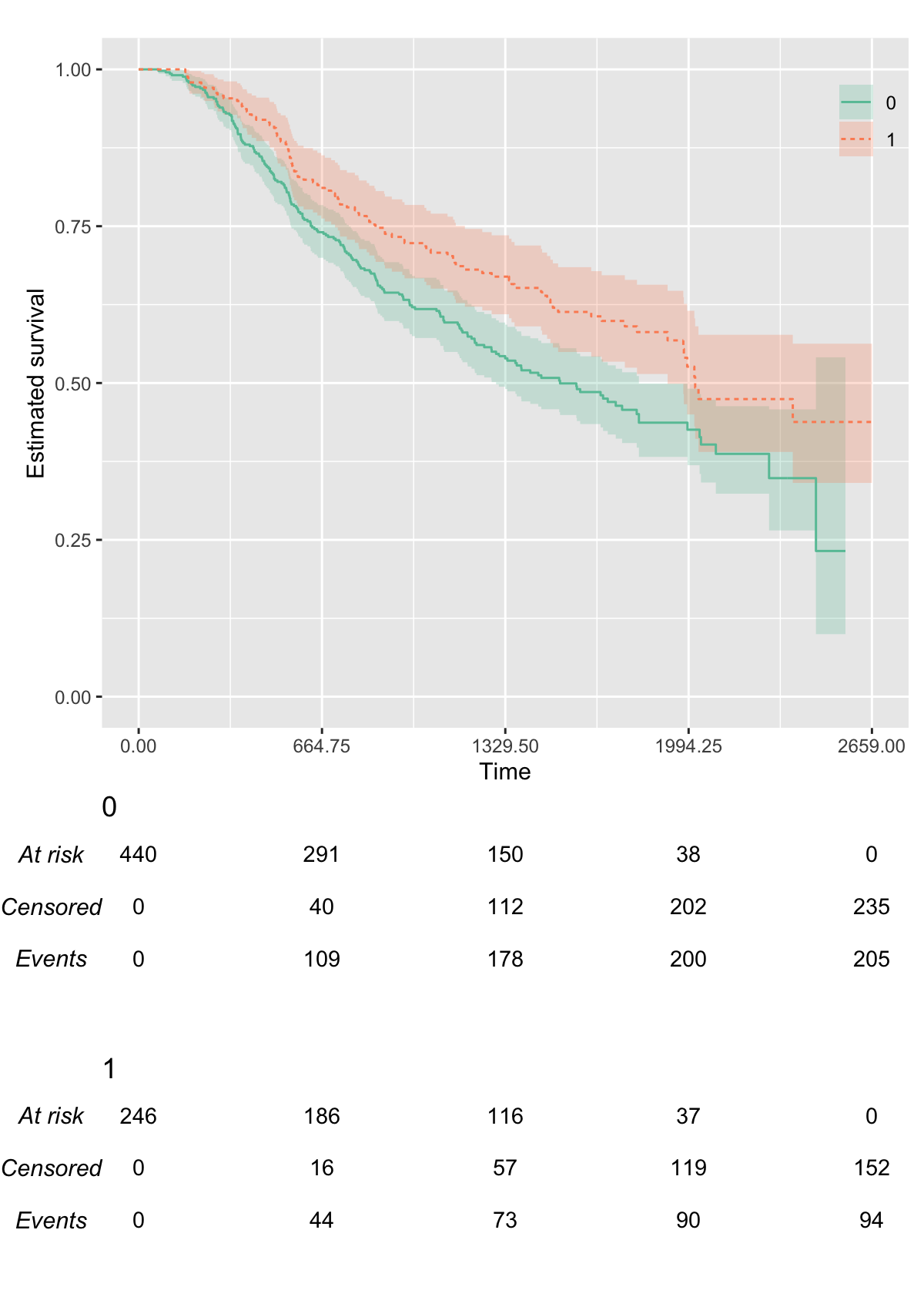We can customise the transparency of the point-wise confidence intervals via the `.alpha` argument:

``KMunicate(fit = fit2, time_scale = ts, .alpha = 0.1)``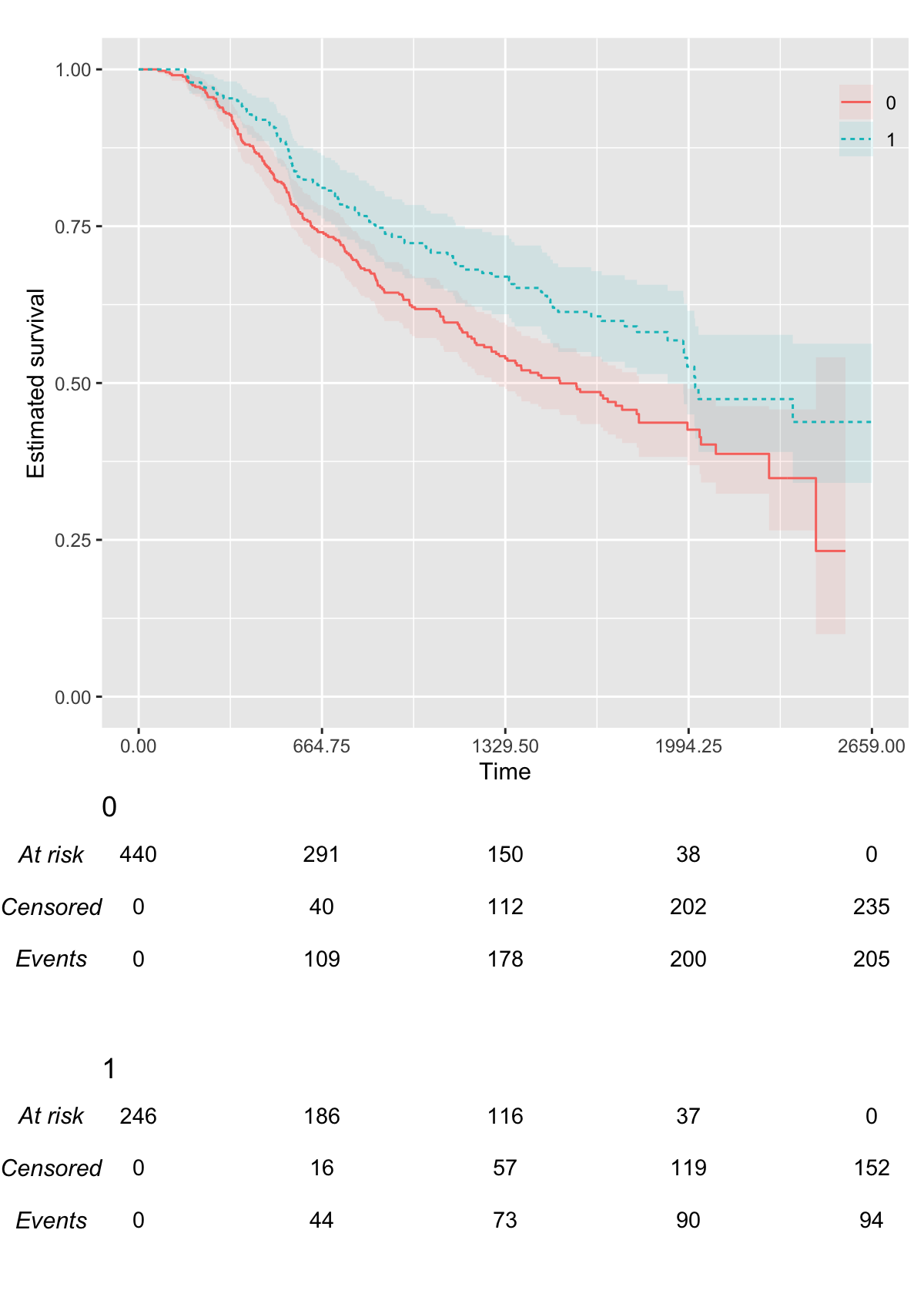We can customise the label of the horizontal axis:

``KMunicate(fit = fit2, time_scale = ts, .xlab = "New Label (Time in Days, Actually)")``